Courses

# Solved Questions - Linear Equations in One Variable Class 8 Notes | EduRev

## Class 8 Mathematics by VP Classes

Created by: Vp Classes

## Class 8 : Solved Questions - Linear Equations in One Variable Class 8 Notes | EduRev

The document Solved Questions - Linear Equations in One Variable Class 8 Notes | EduRev is a part of the Class 8 Course Class 8 Mathematics by VP Classes.
All you need of Class 8 at this link: Class 8

Ques 1: Show that x = 4 is a solution of the equation: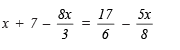Solution: Substituting x = 4 in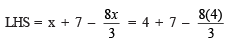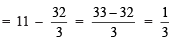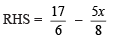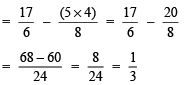Since, LHS = RHS

∴ x = 4 is a solution of the given equation.

Ques 2: Solve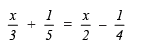Solution: We have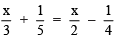LCM of 3, 5, 2 and 4 is 60.

∴ The given equation can be expressed as: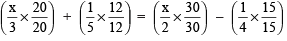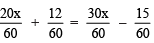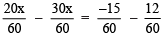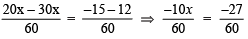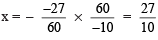Thus, x = 27/10 is the required solution.

Ques 3: Solve for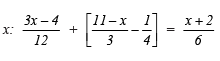Solution: We have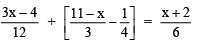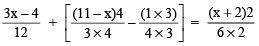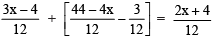⇒ 3x – 4 + 44 – 4x – 3 = 2x + 4

⇒ 3x – 4x – 2x = 4 + 3 – 44 + 4

⇒ 3x – 6x = 11 – 44

⇒ –3x = –33 ⇒ x = 11

Ques 4: Solve for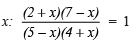Solution: We haveBy cross multiplication, we get:

(2 + x)(7 – x) = (5 – x)(4 + x)

⇒ 2(7 – x) + x(7 – x) = 5(4 + x) – x(4 + x)

⇒ 14 – 2x + 7x – x2 = 20 + 5x – 4x – x2

⇒ –x2 + x2 – 2x + 7x – 5x + 4x = 20 – 14

⇒ –7x + 7x + 4x = 6

⇒  4x = 6 ⇒ x = 6/4 or 3/2

Thus, the solution of the given equation is x = 3/2

Ques 5: A number is such that it is as much greater than 65 as it is less than 91. Find the number.
Solution: Let the number be x.
Since, we have [The number] – 65 = 91 – [The number]

⇒ x – 65 = 91 – x
⇒ x + x = 91 + 65
⇒ 2x = 156

⇒ x = 156/2 = 78

Thus, the required number is 78.

Ques 6: The numerator of a fraction is 2 less than the denominator. If 1 is added to its denominator, it becomes 1/2. Find the fraction.
Solution: Let the denominator of the fraction be x.

∴ Numerator = x – 2

The fraction =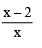Since, it becomes 1/2

When 1 is added to its denominator.

i.e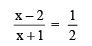By cross multiplication, we have
2(x – 2) = x + 1

⇒ 2x – 4 = x + 1
⇒ 2x – x = 1 + 4
⇒ x = 5

⇒ Fraction =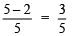Ques 7: After 24 years I shall be 3 times as old as I was 4 years ago. Find my present age.
Solution: Let my present age be x years.

∴ After 24 years, my age will be (x + 24) years.
4 years ago, my age was (x – 4) years.

According to the given condition, we have

(x + 24) = 3(x – 4)

⇒ x + 24 = 3x – 12
⇒ x – 3x = –12 – 24
⇒ –2x = –36

⇒ x = -36/-2 = 18

Thus, my present age is 18 years.

Ques 8: If the sum of two numbers is 30 and their ratio is 2 : 3, then find the numbers.
Solution: Let one of the numbers be x.

∴ The other number = (30 – x)

According to the condition, we have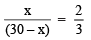[∵ The ratio number is 2 : 3]

⇒ 3x = 2(30 – x) [By cross multiplication]

⇒ 3x = 60 – 2x

⇒ 3x + 2x = 60

⇒ 5x = 60

⇒ x = 60/5 = 12

∴ 30 – x = 30 – 12 = 18

Thus, the required numbers are 12 and 18.

,

,

,

,

,

,

,

,

,

,

,

,

,

,

,

,

,

,

,

,

,

;